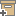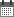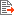Syntax
Search...
Manage
Community:
אסיף מאגר המחקר החקלאי
Determination of rate coefficients for potassium-calcium exchange on vermiculite using a stirred-flow chamberBack to searchPrevious item
Next item
Year:
1995
Authors :
Bar-Tal, Asher
;
.
Feigenbaum, Sala
;
.
Volume :
59
Co-Authors:
Bar-Tal, A.
Eick, M.J.
Feigenbaum, S.
Sparks, D.L.
Fishman, S.
Facilitators :
From page:
760
To page:
765
(
Total pages:
6
)
Abstract:
The objectives of this study were to: (i) develop a mathematical model of the kinetics of an elementary cation-exchange and (ii) determine rate coefficients based on this model for K-Ca exchange on a vermiculite. The kinetics of exchange were described by a second-order mechanistic rate law from which the equilbrium exchange equation was derived. The second-order mechanistic rate law combined with an equation of mass balance describing transport were solved numerically. -from Authors
Note:
Related Files :
soil chemistry
stirred flow method
vermiculite
Related Content
More details
DOI :
Article number:
Affiliations:
Database:
Scopus
Publication Type:
Language:
English
Editors' remarks:
ID:
29236
Last updated date:
02/03/2022 17:27
Creation date:
17/04/2018 00:45Scientific Publication
Determination of rate coefficients for potassium-calcium exchange on vermiculite using a stirred-flow chamber
59
Bar-Tal, A.
Eick, M.J.
Feigenbaum, S.
Sparks, D.L.
Fishman, S.
Determination of rate coefficients for potassium-calcium exchange on vermiculite using a stirred-flow chamber
The objectives of this study were to: (i) develop a mathematical model of the kinetics of an elementary cation-exchange and (ii) determine rate coefficients based on this model for K-Ca exchange on a vermiculite. The kinetics of exchange were described by a second-order mechanistic rate law from which the equilbrium exchange equation was derived. The second-order mechanistic rate law combined with an equation of mass balance describing transport were solved numerically. -from Authors
Scientific Publication
You may also be interested in